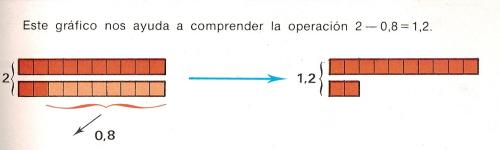4. Subtraction of decimals to printName: ______________________________________  Subject: _______________________ Date: _______

Write the missing word

On the left picture, we have 2 units or 20 decimals and we subtract 8 decimals or 0.8 units. Finally on the right picture, we have 12 decimals or 1.2 units.

2 - 0,8 = 1,2

When we subtract decimals numbers, we must take into account:

Line up the subtrahend (the smaller number) with the minuend (the greater number) vertically so that the decimal points and units all lie on a vertical line.

If the minuend and the subtrahend do not have the same number of digits, we have to add extra zeros to the right of the number so that each number has the same number of digits to the right of the decimal place.

Subtract the numbers as you would whole numbers.

Ā Place the decimal point of the result in line with the other decimal points.

Example:         3             ->      3,00
-  1,25        ->       1,25
_______               ______
1,75        ->       1,75

Remember that the minuend = subtrahend + difference (or result) and check that the subtraction is correct.

Are these decimal numbers in the correct position for the subtraction?

 4,25 -3,128 4,00     -1,83 3,85  -      7 8,450  -  4,128 8,37  -  5,00

Write these numbers on paper and solve the subtractions:

 3,24  -  1,18  = 5 - 4,55 = 12,3 - 9,38 = 7,376 - 5 = 25 - 13,5 =

Convert these expressions into decimal numbers and then subtract them
Example: five MINUS four and five tenths: 5 - 4.5 = 0.5

 Two and seventy-five hundredths  MINUS twenty-five hundredths = Five  MINUS eight thousandths = = Fourteen and six hundredths  MENOS ochenta milķsimas = Fifty MINUS two and three tenths = Twenty-eight hundredths MINUS thirty-two thousandths =

Ask how formulate problemas and then solve them:

 Miguela has 2.50 euros and she spends 1.25 euros on trinkets. How much money does she have? One rope is 1.35 meters and the other is 0.75. How long are the two ropes if we joint them? Esteban had \$ 5.25 and he spent \$ 4. How much money does he have? Last Thursday I walked 1.35 kilometers and last Sunday I walked 2.3 kilometers. How many kilometers do I walk in these two days? A bottle has 1.5 liters and I drink 0.5 liters. How many liters do we have?

| Educational applications | Mathematics |
In Spanish | Interactive  |

«Arturo Ramo GarcĒa.-Record of intellectual property of Teruel (Spain) No 141, of 29-IX-1999
Plaza Playa de Aro, 3, 1║ DO 44002-TERUEL